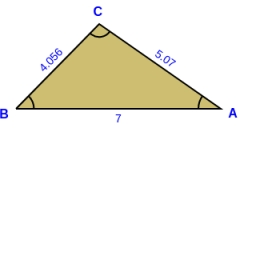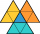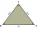# Three sides

Side b is 2 cm longer than side c, side a is 9 cm shorter than side b. The triangle circumference is 40 cm. Find the length of sides a, b, c . .. .

a =  8
b =  17
c =  15

### Step-by-step explanation:

b = 2+c
a = b-9
a+b+c = 40

b-c = 2
a-b = -9
a+b+c = 40

a = 8
b = 17
c = 15

Our linear equations calculator calculates it.Did you find an error or inaccuracy? Feel free to write us. Thank you!Tips to related online calculators
Do you have a system of equations and looking for calculator system of linear equations?

#### You need to know the following knowledge to solve this word math problem:

We encourage you to watch this tutorial video on this math problem:

## Related math problems and questions:

• Sides of triangleTriangle has circumference 42 cm. Side a is 2 times shorter than side b and sice c is 2 cm longer than side a. Determine the sizes of sides of a triangle.
• Triangle - is RT?Triangle has a circumference of 90 cm. Side b is 1 cm longer than c, and side c is 31 cm longer than side a. Calculate the length of sides and determine whether a triangle is a right triangle.
• Ratio of sidesThe triangle has a circumference of 21 cm, and the length of its sides is in a ratio of 6: 5: 3. Find the length of the longest side of the triangle in cm.
• Two similarTwo similar triangles, one has a circumference of 100 cm, the second has sides successively 8 cm, 14 cm, 18 cm longer than the first. Find the lengths of its sides.
• Rectangle - sidesWhat is the perimeter of a rectangle with area 266 cm2 if length of the shorter side is 5 cm shorter than the length of the longer side?
• Triangle ABCIn a triangle ABC side b measure 10 cm less than the side a and side b is half of the side c. Calculate the length of sides if the circumference of the triangle is 42 cm.
• ParallelogramThe perimeter of the parallelogram is 417 cm. The length of one side is 1.7-times longer than the length of the shorter side. What is the length of sides of a parallelogram?
• Rectangles - sidesOne side of the rectangle is 10 cm longer than a second. Shortens longer side by 6 cm and extends shorter by 14 cm increases the rectangle area by 130 cm2. What are the dimensions of the original rectangle?
• HarryHarry Thomson bought a large land in the shape of a rectangle with a circumference of 90 meters. He divided it into three rectangular plots. The shorter side has all three plots of equal length, their longer sides are three consecutive natural numbers. Fi
• Rectangle vs squareOne side of the rectangle is 1 cm shorter than the side of the square, the second side is 3 cm longer than the side of the square. Square and rectangle have the same content. Calculate the length of the sides of a square and a rectangle.
• RT perimeterThe leg of the rectangular triangle is 7 cm shorter than the second leg and 8 cm shorter than the hypotenuse. Calculate the triangle circumference.
• Sides of triangleTriangle circumference with two identical sides is 117cm. The third side measures 44cm. How many cms do you measure one of the same sides?
• ParallelogramSide a of parallelogram is twice longer than side b. Its circumference is 78 dm. Calculate the length of the sides of a parallelogram.
• Arm and baseThe isosceles triangle has a circumference of 46 cm. Calculate its area if the arm is 5 cm longer than the base.
• Triangle and its heightsCalculate the length of the sides of the triangle ABC, if va=5 cm, vb=7 cm and side b is 5 cm shorter than side a.
• The sidesThe sides of the rectangle are in a ratio of 3: 5 and its circumference measures 72 cm. Calculate: a) the size of both sides of the rectangle b) the area of the rectangle c) the length of the diagonals
• Right triangle eq2Hypotenuse of a right triangle is 9 cm longer than one leg and 8 cm longer than the second leg. Determine the circumference and area of a triangle.